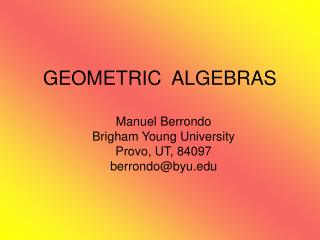DownloadDownload PresentationGEOMETRIC ALGEBRAS

# GEOMETRIC ALGEBRAS

Télécharger la présentation## GEOMETRIC ALGEBRAS

- - - - - - - - - - - - - - - - - - - - - - - - - - - E N D - - - - - - - - - - - - - - - - - - - - - - - - - - -
##### Presentation Transcript

1. GEOMETRIC ALGEBRAS Manuel Berrondo Brigham Young University Provo, UT, 84097 berrondo@byu.edu

2. Physical Applications: • Mechanics: Foucault pendulum • Electro-magnetostatics • Dispersion and diffraction E.M. • Quantum Mechanics: spin precession • Field Theory: Dirac equation • (General Relativity )

3. Examples of geometric algebras • Complex Numbers G0,1= C • Hamilton Quaternions G0,2= H • Pauli Algebra G3 • Dirac Algebra G1,3

4. Extension of the vector space • inverse of a vector: • reflections, rotations, Lorentz transform. • integrals: Cauchy (≥ 2 d), Stokes’ theorem Based on the idea of MULTIVECTORS: and including lengths and angles:

5. Geometric or matrix product

6. + closure commutativity associativity zero negative • closure commutativity associativity unit reciprocal and + distributivity Algebraic Properties of R Vector spaces : linear combinations

7. Algebras • include metrics: • define geometricproduct FIRST STEP: Inverse of a vector a≠ 0 a-1 0 2 a 1

8. 3d:ê1 ê2 ê3 êi •êk = i k êi-1= êi Euclidian 4d: 0 1 2 3 μ•ν= gμν (1,-1,-1,-1) Minkowski Orthonormal basis Example: (ê1+ ê2)-1 = (ê1+ ê2)/2 1

9. Electrostatics: method of images w r • - q • ql • q • q Plane: charge -q atcê1, image (-q) at -cê1 Sphere radius a, charge q atbê1, find image (?)

10. Choose scales for r and w:

11. SECOND STEP: EULER FORMULAS In 3-d: given that In general, rotating A about θ k:

12. Example 1: Lorentz Equation q B = B k

13. Example 2: Foucault Pendulum l S Coriolis • mg

14. THIRD STEP: ANTISYM. PRODUCT b a sweep b sweep a • anticommutative • associative • distributive • absolute value => area

15. Geometric or matrix product • non commutative • associative • distributive • closure: • extend vector space • unit = 1 • inverse (conditional)

16. Examples of Clifford algebras

17. G2: R R2 I R C = even algebra = spinors

18. I e2 • z a C isomorphic to R2 1 e1 • z* z = ê1a ê1z = a Reflection: z  z* a a’ = ê1z* = ê1a ê1 In general, a’ = n a n, with n2 = 1

19. a’ e2 φ Rotations in R2 Euler: a e1

20. Inverse of multivector in G2Conjugate: Generalizing

21. G3 R3 iR R H = R + i R3= = even algebra = spinors iR3

22. Geometric product in G3 : Rotations: e P/2 generates rotations with respect to planedefined by bivector P

23. Spinors σ, Pauli matrices

24. Interaction: spin with magnetic field B • Pauli’s quantum Hamiltonian with A =vector potential p  p – q A, minimal coupling, magnetic moment : μ = S = ħσ/2

25. Spin precession with uniform B : In G3, spinor (quaternion) Ψ, Solution in G3: Spin precesses on plane i B withangularfreq. independent of ħ !

26. Inverse of multivector in G3defining Clifford conjugate: • Generalizing:

27. Special relativity and paravectors in G3 • Paravector p = p0 + p Examples of paravectors:

28. Lorentz transformations Transform the paravector p = p0 + p = p0 + p=+ p┴ into: B p B = B2 (p0 + p=) + p┴ R p R† = p0 + p= + R2 p┴

29. Geometric Calculus (3d) • is a vector differential operator acting on:  (r) – scalar field E(r) – vector field

30. First order Green Functions Euclidian spaces : is solution of

31. Maxwell’s Equation • Maxwell’s multivector F = E +i c B • current density paravector J = (ε0c)-1(cρ + j) • Maxwell’s equation:

32. Electrostatics Gauss’s law. Solution using first order Green’s function: Explicitly:

33. Magnetostatics Ampère’s law. Solution using first order Green’s function: Explicitly:

34. Fundamental Theorem of Calculus • Euclidian case : dkx = oriented volume, e.g. ê1 ê2 ê3 = i dk-1x = oriented surface, e.g. ê1 ê2 = iê3 F(x)– multivector field, V – boundary of V

35. Example: divergence theorem • d3x = i d, where d = |d3 x| • d2 x = i nda , where da = |d2 x| • F = v(r)

36. Green’s Theorem (Euclidian case ) • where the first order Green function is:

37. Cauchy’s theorem in n dimensions • particular case : F = f y f = 0 where f (x) is a monogenic multivectorial field: f = 0

38. Inverse of differential paravectors: Helmholtz: Spherical wave (outgoing or incoming)

39. Electromagnetic diffraction • First order Helmholtz equation: • Exact Huygens’ principle: In the homogeneous case J = 0

40. 6 bivectors split into two groups: êi = i0 and I êk = ij

41. Dirac Equation in G1,3 • Vector in Minkowski space : • Quantization:

42. Propagator – first order Green function • The propagator represents the conditional probability amplitude • Fourier transform :

43. Including external field A, Dirac equation is written as: • and the propagator: includes the vacuum polarization term!